Courses
Courses for Kids
Free study material
Free LIVE classes
More

# Equal AnglesLIVE
Join Vedantu’s FREE Mastercalss

## Introduction to Angles

An angle is a figure in Plane Geometry generated by two rays or lines that share a common endpoint. "Angle" comes from the Latin word "angulus," which means "corner." The angle in the plane is present in two forms: a positive angle and a negative angle. A positive angle is formed when the angle is rotated counterclockwise. A negative angle is one that is measured in a clockwise direction.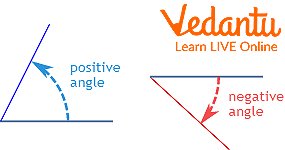Types of Angle

## What are Angles?

When two rays arise from the same spot, an angle is generated. These rays form an angle known as the arms of the angle, and their origin is known as the vertex of the angle.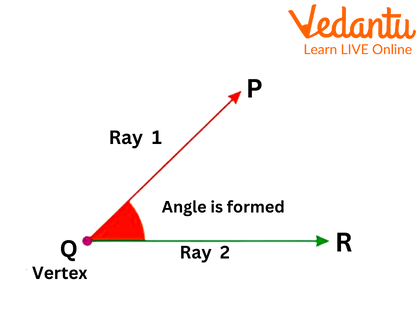Angle Formed by Two Rays

An angle is denoted by the symbol $\angle$. The created angle is indicated by $\angle$PQR in the diagram above. $\angle$RQP is another way to represent the same angle. The degree is used to measure angles.

The above two rays may be combined in a variety of ways to generate the many sorts of angles in geometry.

## Types of Angles

Based on the degree of the angle formed there are different types of angles.

• Zero Angle – The angle formed is zero degrees.

• Acute Angle – The angle formed is greater than zero and less than $90^\circ$.

• Right Angle – The angle formed is $90^\circ$.

• Obtuse Angle – The angle formed is greater than $90^\circ$and less than $180^\circ$.

• Straight Angle – The angle is at $180^\circ$and appears like a straight line.

• Reflex Angle – The angle is greater than $180^\circ$but less than $360^\circ$.

• Complete Angle – The angle which is of $360^\circ$is the complete angle.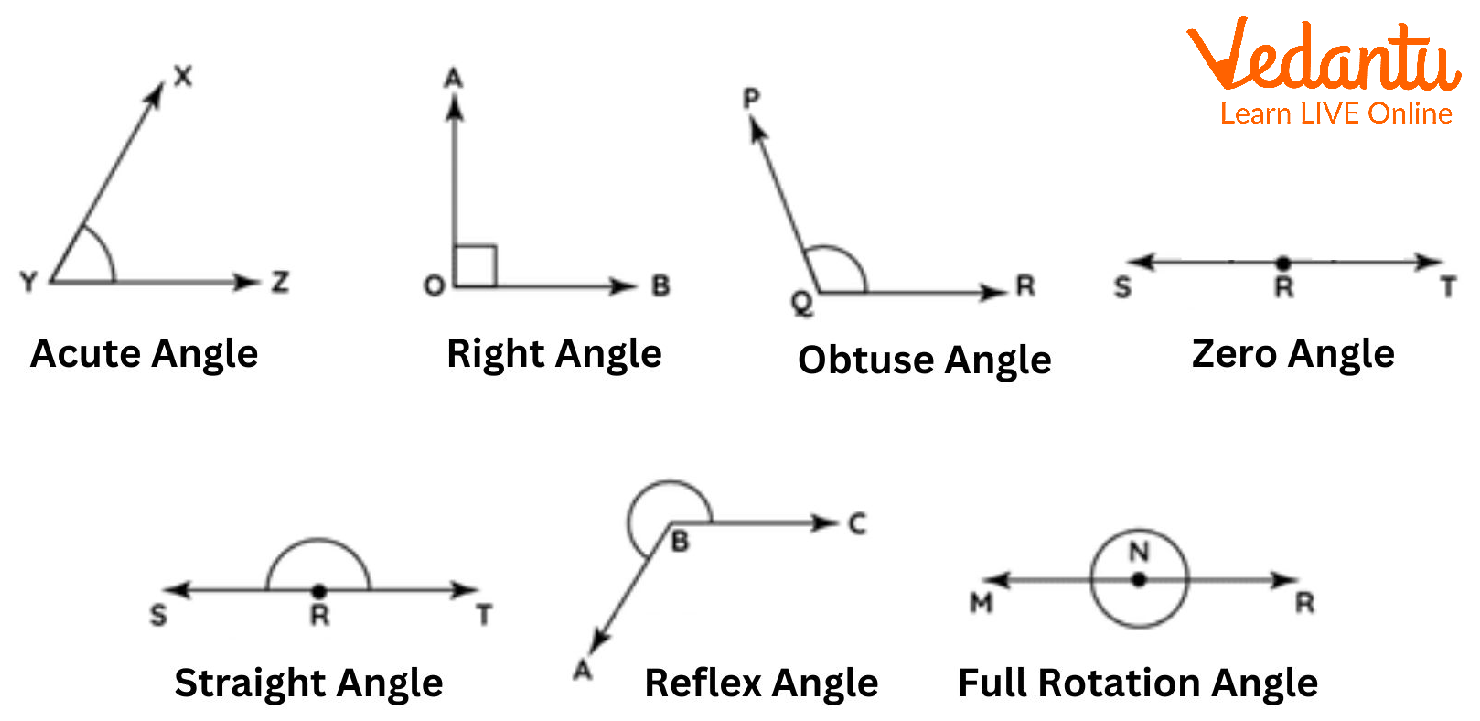Types of Angles Based on the Degree of Angle Formed

## Angles Based on Their Pairing

Based on the pairing of two angles there are different types of angles.

• Complementary Angles – When two angles add up to form $90^\circ$then it is called complementary angles. For example, if we add $30^\circ$and $60^\circ$ we get $90^\circ$.

• Supplementary Angles – When two angles add up to form $180^\circ$then we call it a supplementary angle. For example, if we add $110^\circ$and $70^\circ$we will get $180^\circ$.

• Linear Angles – Two angles are termed to be linear if they are produced by two intersecting lines. Their total is always $180^\circ$.

• Adjacent Angles – Adjacent angles are two angles that share a vertex and a side but do not intersect.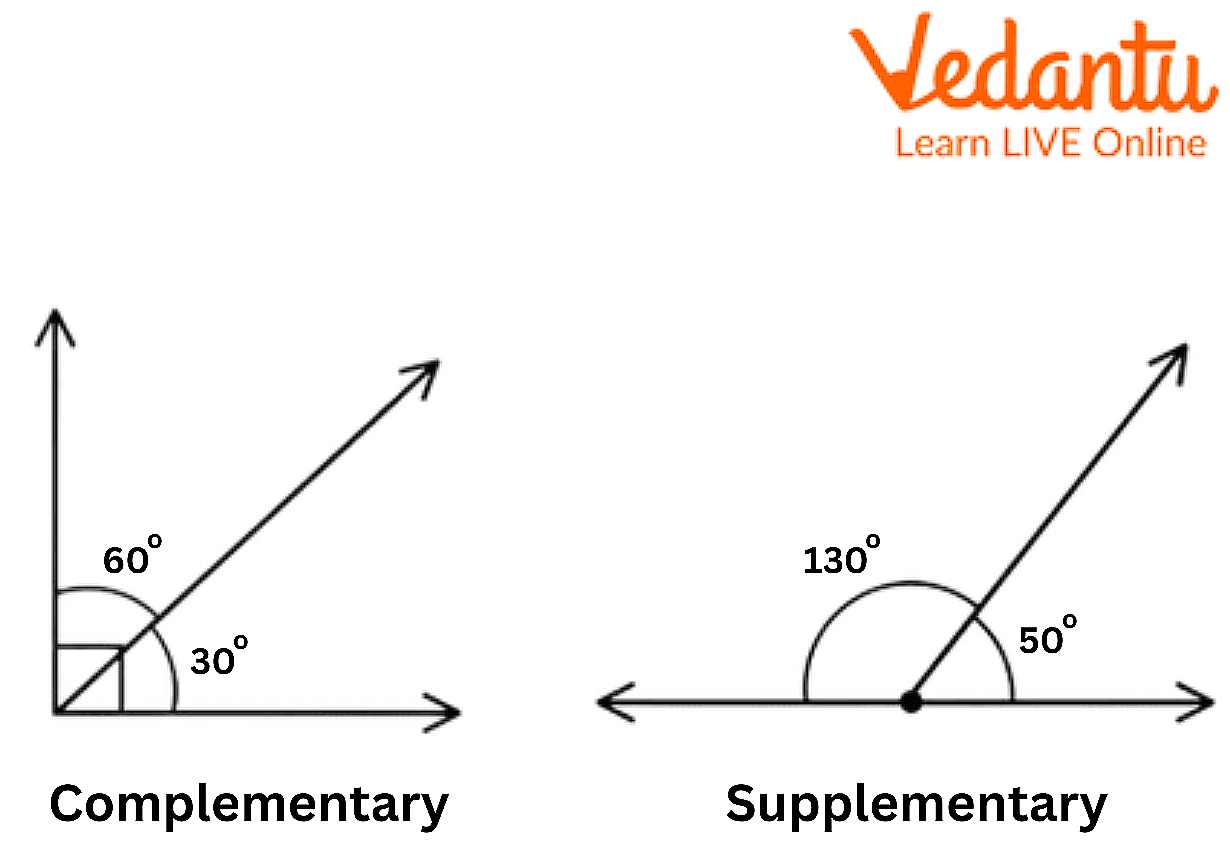Complementary and Supplementary Angles

## Construction of Angles

To construct the angle you need to follow these simple steps.

Step 1: Draw an OA line segment.

Step 2: Set the protractor's center at point O.

Step 3: Working clockwise from point A, mark a point at 50 degrees by glancing at the protractor's outer circle. Make this point B.

The $\angle$BOA represents the needed $50^\circ$ angle.

For the construction of equal angles, you need to use a compass. The method of its construction is bit complex and would be taught to you in higher classes.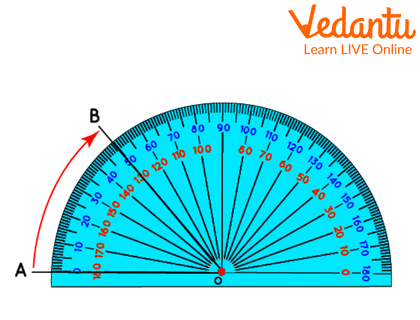Construction of Angle

## Conclusion

Angle is constructed by the two line segments who have a common end-point. Angles can be formed by two-line segments using a mathematical tool named a protractor. Two equal supplementary angles will result to form a whole circle or whole angle of $360^\circ$. The different types of angles formed can be calculated using the simple operations of addition and subtraction.

## Sample Questions

1. The angle complementary to $40^\circ$ is

a. $30^\circ$

b. $40^\circ$

c. $50^\circ$

d. $60^\circ$

Ans: $50^\circ$

Explanation: For angles to be complementary their sum should be equal to 90.

$\begin{array}{l}40 + x = 90\\x = 90 - 40\\x = 50^\circ \end{array}$

2. The angle supplementary to $60^\circ$ is

a. $160^\circ$

b. $140^\circ$

c. $120^\circ$

d. $100^\circ$

Ans: $120^\circ$

Explanation: For angles to be supplementary their sum should be equal to 180.

$\begin{array}{l}60 + x = 180^\circ \\x = 180^\circ \ - 60^\circ \\x = 120^\circ \end{array}$

3. Find the measure of all angles of an equilateral triangle?

a. $60^\circ$

b. $10^\circ$

c. $120^\circ$

d. $100^\circ$

Ans: $60^\circ$

Explanation: The angles in the equilateral triangle are also equal. The sum of all the angles in a triangle is 180.

$\begin{array}{l}3x = 180\\x = \dfrac{{180}}{3} = 60^\circ \end{array}$

So, in an equilateral triangle, each angle is 60 degrees.

Last updated date: 30th Sep 2023
Total views: 67.8k
Views today: 1.67k

## FAQs on Equal Angles

1. Will two acute angles be supplementary?

No, because two angles are supplementary, they are either both right angles or one angle is acute and the other is obtuse. Because the total of two acute angles is always less than $180^\circ$, two acute angles can never be supplementary angles.

2. Could two obtuse angles be supplementary?

No, because two angles are supplementary, they are either both right angles or one is acute and the other is obtuse. Because the total of two obtuse angles is always more than $180^\circ$, two obtuse angles can't be supplementary angles.

3. How do you determine angles?

An angle can be identified by seeing the figure or measuring the angles using a protractor.

4. Which angle has the greatest magnitude?

A full angle is one whose length is precisely equal to 360. It is also known as a complete angle. As a result, the total angle is the greatest.

5. What are transversal angles?

A transversal is a line that crosses two or more lines at distinct locations. The 8 angles formed are the transversal angles.